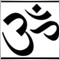# Close at percentage loss[Deleted]

I have the necessary code that allows open orders to close once the predefined percentage of profit is reached (below). I can't find any command in the dictionary that would perform the same function to close at percentage loss. CloseLossPercentage is not a recognised command.

bool hasProfit() {
int cnt;
int total=OrdersTotal();
double totalProfit = 0;
for(cnt=0;cnt<total;cnt++) {
totalProfit += OrderProfit();
}
if (((AccountBalance() * closeProfitPercentage) / 100) < totalProfit)
return (true);
return (false);
}5300

patrick007:

I have the necessary code that allows open orders to close once the predefined percentage of profit is reached (below). I can't find any command in the dictionary that would perform the same function to close at percentage loss.

There is no such command. You check if OrderProfit() or AccountEquity() is where you want. Then you use OrderClose() to close your Order(s).[Deleted]
ubzen:
There is not such command.17410

patrick007:

I have the necessary code that allows open orders to close once the predefined percentage of profit is reached (below). I can't find any command in the dictionary that would perform the same function to close at percentage loss. CloseLossPercentage is not a recognised command.

The code to do what you want is almost identical . . .  a percentage loss is just a negative percentage profit.[Deleted]

You can maybe use a mathematic formula:

Example:

```double X= 30; //(30 is an exemple !) The % you want

if (AccountEquity()==(accountequity -(account equity * X/100))

OrderClose;```5300

Kane59: You can maybe use a mathematic formula: Example:
Nice example. I personally would feel more comfortable with <= instead of == just in case price dips below that number on ticks. Also comparing doubles with = sign can be tricky business in mql4. Here's my example for what its worth.[Deleted]
RaptorUK:
The code to do what you want is almost identical . . .  a percentage loss is just a negative percentage profit.

Thank you for your reply. Are you saying simply add the bool again, but the line where the profit percentage is should have - before the number?[Deleted]
Kane59:

You can maybe use a mathematic formula:

Example:17410

patrick007:
Thank you for your reply. Are you saying simply add the bool again, but the line where the profit percentage is should have - before the number?
Ideally I would rewrite your function to return a bool when given the value to check the profit against . . .  for example if you want to check for a profit of 10%   use  hasProfit(10)  to check for a loss of 10% use  hasProfit(-10) . .  .  it's just simple mathematics . . .[Deleted]
ubzen:

Nice example. I personally would feel more comfortable with <= instead of == just in case price dips below that number on ticks. Also comparing doubles with = sign can be trick business in mql4.

Yes , i agree :) <= will be more efficient for this example.[Deleted]
RaptorUK:
Ideally I would rewrite your function to return a bool when given the value to check the profit against . . .  for example if you want to check for a profit of 10%   use  hasProfit(10)  to check for a loss of 10% use  hasProfit(-10) . .  .  it's just simple mathematics . . .

Appreciate it. Logic can not be bought or sold. Some possess it in greater quantities and qualities than others :) I'll get my coat. Thanks.